## TyroCity

• Nucleus become stable only for a certain number of protons and neutrons the instability exist is the nucleus if attractive force (nuclear force) or repulsive force (electrostatic force) dominates one another.
• The instability in the nucleus leads disintegration spontaneously. It corresponds to the emission of alpa-particle, beta-particle or gamma rays.
• If alpha-particles are emitted nucleus loses 2 units of its positive charge and 4 units of its mass (2He4).
• IF bea-particles are emitted nucleus gains 1 unit of +ve charge and 0 units of its mass
• The no. of atoms present in the radioactive substance depends upon the rate of disintegration. According to the following relation.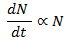It states that “rate of disintegration is directly proportional to the number of atoms present”. This is known as law of Radioactive decay as well.
Let us consider N0 be the total no. of atoms present. dN/dt be the rate of disintegration of atoms. N be the no. of atoms present after time ‘t’.
According to decay law,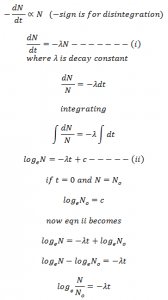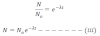his equation gives the no. of atoms present after certain interval of time.
This equation can also be expressed in graph as: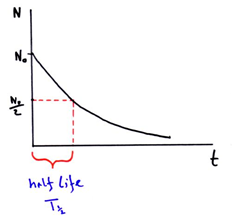From equation (iii) we get,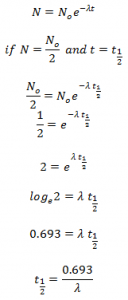This expression gives the half size of the radioactive substance in terms of decay constant. Here, half life is reciprocal of decay constant.
Hence, half life of a radioactive substance is defined as the time period during which half of the no. of atoms of the substance remains un-decayed.